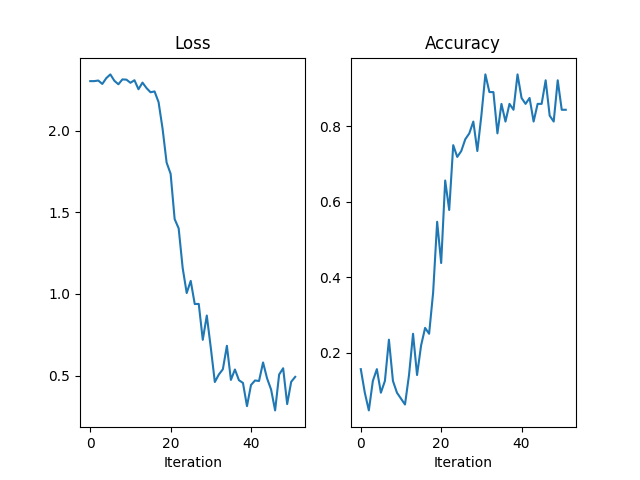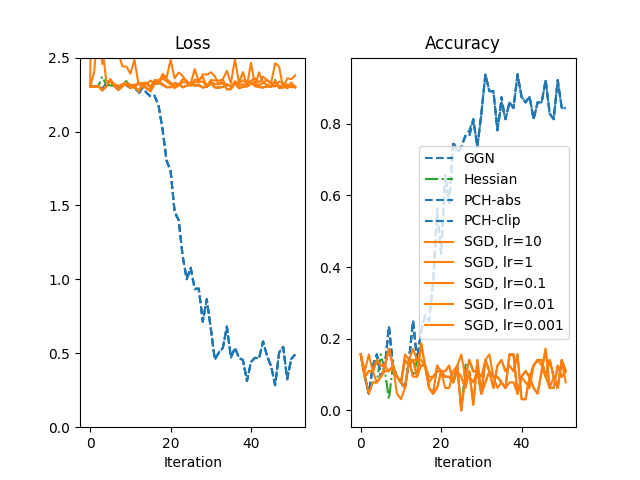# Matrix-free second-order optimization

This example walks you through a second-order optimizer that uses the conjugate gradient (CG) method and matrix-free multiplication with the block diagonal of different curvature matrices to solve for the Newton step.

The optimizer is tested on the classic MNIST example from PyTorch. In particular, we will use a model that suffers from the vanishing gradient problem and is hence difficult to optimizer for gradient descent. Second-order methods are less affected by that issue and can train these models, as they rescale the gradient according to the local curvature.

A local quadratic model of the loss defined by a curvature matrix $$C(x_t)$$ (the Hessian, generalized Gauss-Newton, or other approximations)is minimized by taking the Newton step

$x_{t+1} = x_t - \gamma (C(x_t) + \lambda I)^{-1} g(x_t),$

where

$\begin{split}\begin{array}{ll} x_t: & \text{parameters of the model} \\ g(x_t): & \text{gradient} \\ C(x_t): & \text{curvature of the local quadratic model at x_t} \\ \lambda: & \text{damping parameter} \\ \gamma: & \text{step-size} \\ \end{array}\end{split}$

Let’s get the imports, configuration and some helper functions out of the way first. Notice that we are choosing a net with many sigmoids to make it hard to train for SGD.

Note

Larger batch sizes are usually recommended for second-order methods. However, the memory constraints imposed by the architecture used to build this example restrict us to rather small values.

import math

import matplotlib.pyplot as plt
import torch

from backpack import backpack, extend, extensions

BATCH_SIZE = 64
LR = 0.1
DAMPING = 1e-2
CG_TOL = 0.1
CG_ATOL = 1e-6
CG_MAX_ITER = 20
MAX_ITER = 50
PRINT_EVERY = 10
DEVICE = torch.device("cuda:0" if torch.cuda.is_available() else "cpu")

torch.manual_seed(0)

def make_model():
torch.nn.Conv2d(1, 10, 5, 1),
torch.nn.Sigmoid(),
torch.nn.MaxPool2d(2, 2),
torch.nn.Conv2d(10, 20, 5, 1),
torch.nn.Sigmoid(),
torch.nn.MaxPool2d(2, 2),
torch.nn.Flatten(),
torch.nn.Linear(4 * 4 * 20, 50),
torch.nn.Sigmoid(),
torch.nn.Linear(50, 10),
)

model = make_model().to(DEVICE)

loss_function = torch.nn.CrossEntropyLoss().to(DEVICE)

def get_accuracy(output, targets):
"""Helper function to print the accuracy"""
predictions = output.argmax(dim=1, keepdim=True).view_as(targets)
return predictions.eq(targets).float().mean().item()


## Writing the optimizer

To compute the update, we need access to the curvature matrix in form of matrix-vector products. We can then solve the linear system implied by the Newton step with CG,

$(C(x_t) + \lambda I) v = - g(x_t),$

and perform the update

$x_{t+1} = x_t - \gamma v,$

for every parameter.

Here is the optimizer. At its core is a simple implementation of CG that will iterate until the residual norm decreases a certain threshold (determined the atol and tol arguments), or exceeds a maximum budget (maxiter).

class CGNOptimizer(torch.optim.Optimizer):
def __init__(
self,
parameters,
bp_extension,
lr=0.1,
damping=1e-2,
maxiter=100,
tol=1e-1,
atol=1e-8,
):
super().__init__(
parameters,
dict(
lr=lr,
damping=damping,
maxiter=maxiter,
tol=tol,
atol=atol,
savefield=bp_extension.savefield,
),
)
self.bp_extension = bp_extension

def step(self):
for group in self.param_groups:
for p in group["params"]:
damped_curvature = self.damped_matvec(
p, group["damping"], group["savefield"]
)

direction, info = self.cg(
damped_curvature,
maxiter=group["maxiter"],
tol=group["tol"],
atol=group["atol"],
)

def damped_matvec(self, param, damping, savefield):
curvprod_fn = getattr(param, savefield)

def matvec(v):
v = v.unsqueeze(0)
result = damping * v + curvprod_fn(v)
return result.squeeze(0)

return matvec

@staticmethod
def cg(A, b, x0=None, maxiter=None, tol=1e-5, atol=1e-8):
r"""Solve :math:Ax = b for :math:x using conjugate gradient.

The interface is similar to CG provided by :code:scipy.sparse.linalg.cg.

The main iteration loop follows the pseudo code from Wikipedia:

Parameters
----------
A : function
Function implementing matrix-vector multiplication by A.
b : torch.Tensor
Right-hand side of the linear system.
x0 : torch.Tensor
Initialization estimate.
atol: float
Absolute tolerance to accept convergence. Stop if
:math:|| A x - b || < atol
tol: float
Relative tolerance to accept convergence. Stop if
:math:|| A x - b || / || b || < tol.
maxiter: int
Maximum number of iterations.

Returns
-------
x (torch.Tensor): Approximate solution :math:x of the linear system
info (int): Provides convergence information, if CG converges info
corresponds to numiter, otherwise info is set to zero.
"""
maxiter = b.numel() if maxiter is None else min(maxiter, b.numel())
x = torch.zeros_like(b) if x0 is None else x0

# initialize parameters
r = (b - A(x)).detach()
p = r.clone()
rs_old = (r**2).sum().item()

# stopping criterion
norm_bound = max([tol * torch.norm(b).item(), atol])

def converged(rs, numiter):
"""Check whether CG stops (convergence or steps exceeded)."""
norm_converged = norm_bound > math.sqrt(rs)
info = numiter if norm_converged else 0
iters_exceeded = numiter > maxiter
return (norm_converged or iters_exceeded), info

# iterate
iterations = 0
while True:
Ap = A(p).detach()

alpha = rs_old / (p * Ap).sum().item()
r.sub_(Ap, alpha=alpha)
rs_new = (r**2).sum().item()
iterations += 1

stop, info = converged(rs_new, iterations)
if stop:
return x, info

p.mul_(rs_new / rs_old)
rs_old = rs_new


## Running and plotting

Let’s try the Newton-style CG optimizer with the generalized Gauss-Newton (GGN) as curvature matrix.

After extend-ing the model and the loss function and creating the optimizer, we have to add the curvature-matrix product extension to the with backpack(...) context in a backward pass, such that the optimizer has access to the GGN product. The rest is just a canonical training loop which logs and visualizes training loss and accuracy.

model = extend(model)
loss_function = extend(loss_function)
optimizer = CGNOptimizer(
model.parameters(),
extensions.GGNMP(),
lr=LR,
damping=DAMPING,
maxiter=CG_MAX_ITER,
tol=CG_TOL,
atol=CG_ATOL,
)

losses = []
accuracies = []
for batch_idx, (x, y) in enumerate(mnist_loader):

x, y = x.to(DEVICE), y.to(DEVICE)
outputs = model(x)
loss = loss_function(outputs, y)

with backpack(optimizer.bp_extension):
loss.backward()

optimizer.step()

# Logging
losses.append(loss.detach().item())
accuracies.append(get_accuracy(outputs, y))

if (batch_idx % PRINT_EVERY) == 0:
print(
"Iteration %3.d/%3.d " % (batch_idx, MAX_ITER)
+ "Minibatch Loss %.5f  " % losses[-1]
+ "Accuracy %.5f" % accuracies[-1]
)

if MAX_ITER is not None and batch_idx > MAX_ITER:
break

fig = plt.figure()

axes.plot(losses)
axes.set_title("Loss")
axes.set_xlabel("Iteration")

axes.plot(accuracies)
axes.set_title("Accuracy")
axes.set_xlabel("Iteration")Iteration   0/ 50 Minibatch Loss 2.30490  Accuracy 0.15625
Iteration  10/ 50 Minibatch Loss 2.29479  Accuracy 0.07812
Iteration  20/ 50 Minibatch Loss 1.73662  Accuracy 0.43750
Iteration  30/ 50 Minibatch Loss 0.66946  Accuracy 0.82812
Iteration  40/ 50 Minibatch Loss 0.44161  Accuracy 0.87500
Iteration  50/ 50 Minibatch Loss 0.46170  Accuracy 0.84375

Text(0.5, 23.52222222222222, 'Iteration')


## Vanishing gradients: comparison with SGD

By intention, we chose a model that is different to optimize with gradient descent due to the large number of sigmoids that reduce the gradient signal in backpropagation.

To verify that, let’s compare the Newton optimizer for different curvatures with SGD. SGD is run for a large range of learning rates lr ∈ [10, 1, 0.1, 0.01, 0.001].

The performance of CG-Newton versus SGD is shown below (using a somewhat simplified color scheme to simplify the visualization).

def make_cgn_optimizer_fn(extension):
def optimizer_fn(model):
return CGNOptimizer(
model.parameters(),
extension,
lr=LR,
damping=DAMPING,
maxiter=CG_MAX_ITER,
tol=CG_TOL,
atol=CG_ATOL,
)

return optimizer_fn

curvatures = [
extensions.GGNMP(),
extensions.HMP(),
extensions.PCHMP(modify="abs"),
extensions.PCHMP(modify="clip"),
]

labels = [
"GGN",
"Hessian",
"PCH-abs",
"PCH-clip",
]

optimizers = []
for curvature in curvatures:
optimizers.append(make_cgn_optimizer_fn(curvature))

def make_sgd_optimizer_fn(lr):
def optimizer_fn(model):

return optimizer_fn

sgd_lrs = [
10,
1,
0.1,
0.01,
0.001,
]

for lr in sgd_lrs:
optimizers.append(make_sgd_optimizer_fn(lr))
labels.append("SGD, lr={}".format(lr))

def train(optim_fn):
torch.manual_seed(0)

model = make_model().to(DEVICE)
loss_function = torch.nn.CrossEntropyLoss().to(DEVICE)

optimizer = optim_fn(model)
need_backpack = isinstance(optimizer, CGNOptimizer)

if need_backpack:
model = extend(model)
loss_function = extend(loss_function)

losses = []
accuracies = []
for batch_idx, (x, y) in enumerate(mnist_loader):

x, y = x.to(DEVICE), y.to(DEVICE)
outputs = model(x)
loss = loss_function(outputs, y)

if need_backpack:
with backpack(optimizer.bp_extension):
loss.backward()
else:
loss.backward()

optimizer.step()

# Logging
losses.append(loss.detach().item())
accuracies.append(get_accuracy(outputs, y))

if (batch_idx % PRINT_EVERY) == 0:
print(
"Iteration %3.d/%3.d " % (batch_idx, MAX_ITER)
+ "Minibatch Loss %.5f  " % losses[-1]
+ "Accuracy %.5f" % accuracies[-1]
)

if MAX_ITER is not None and batch_idx > MAX_ITER:
break

return losses, accuracies

fig = plt.figure()

axes.set_title("Loss")
axes.set_ylim(0, 2.5)
axes.set_xlabel("Iteration")

axes.set_title("Accuracy")
axes.set_xlabel("Iteration")

for optim_fn, label in zip(optimizers, labels):
print(label)
losses, accuracies = train(optim_fn)

if "SGD" in label:
axes.plot(losses, "-", color="tab:orange", label=label)
axes.plot(accuracies, "-", color="tab:orange", label=label)
elif "Hessian" in label:
axes.plot(losses, "-.", color="tab:green", label=label)
axes.plot(accuracies, "-.", color="tab:green", label=label)
else:
axes.plot(losses, "--", color="tab:blue", label=label)
axes.plot(accuracies, "--", color="tab:blue", label=label)

plt.legend()GGN
Iteration   0/ 50 Minibatch Loss 2.30490  Accuracy 0.15625
Iteration  10/ 50 Minibatch Loss 2.29479  Accuracy 0.07812
Iteration  20/ 50 Minibatch Loss 1.73662  Accuracy 0.43750
Iteration  30/ 50 Minibatch Loss 0.66946  Accuracy 0.82812
Iteration  40/ 50 Minibatch Loss 0.44161  Accuracy 0.87500
Iteration  50/ 50 Minibatch Loss 0.46170  Accuracy 0.84375
Hessian
Iteration   0/ 50 Minibatch Loss 2.30490  Accuracy 0.15625
Iteration  10/ 50 Minibatch Loss 2.31134  Accuracy 0.07812
Iteration  20/ 50 Minibatch Loss 2.30426  Accuracy 0.10938
Iteration  30/ 50 Minibatch Loss 2.32674  Accuracy 0.10938
Iteration  40/ 50 Minibatch Loss 2.33985  Accuracy 0.09375
Iteration  50/ 50 Minibatch Loss 2.29731  Accuracy 0.10938
PCH-abs
Iteration   0/ 50 Minibatch Loss 2.30490  Accuracy 0.15625
Iteration  10/ 50 Minibatch Loss 2.29477  Accuracy 0.07812
Iteration  20/ 50 Minibatch Loss 1.73108  Accuracy 0.43750
Iteration  30/ 50 Minibatch Loss 0.66395  Accuracy 0.82812
Iteration  40/ 50 Minibatch Loss 0.44050  Accuracy 0.87500
Iteration  50/ 50 Minibatch Loss 0.45998  Accuracy 0.84375
PCH-clip
Iteration   0/ 50 Minibatch Loss 2.30490  Accuracy 0.15625
Iteration  10/ 50 Minibatch Loss 2.29477  Accuracy 0.07812
Iteration  20/ 50 Minibatch Loss 1.73137  Accuracy 0.43750
Iteration  30/ 50 Minibatch Loss 0.66714  Accuracy 0.82812
Iteration  40/ 50 Minibatch Loss 0.44211  Accuracy 0.87500
Iteration  50/ 50 Minibatch Loss 0.46250  Accuracy 0.84375
SGD, lr=10
Iteration   0/ 50 Minibatch Loss 2.30490  Accuracy 0.15625
Iteration  10/ 50 Minibatch Loss 2.39131  Accuracy 0.03125
Iteration  20/ 50 Minibatch Loss 2.48565  Accuracy 0.10938
Iteration  30/ 50 Minibatch Loss 2.40048  Accuracy 0.09375
Iteration  40/ 50 Minibatch Loss 2.46472  Accuracy 0.09375
Iteration  50/ 50 Minibatch Loss 2.35458  Accuracy 0.14062
SGD, lr=1
Iteration   0/ 50 Minibatch Loss 2.30490  Accuracy 0.15625
Iteration  10/ 50 Minibatch Loss 2.29233  Accuracy 0.07812
Iteration  20/ 50 Minibatch Loss 2.30193  Accuracy 0.10938
Iteration  30/ 50 Minibatch Loss 2.32728  Accuracy 0.04688
Iteration  40/ 50 Minibatch Loss 2.33464  Accuracy 0.09375
Iteration  50/ 50 Minibatch Loss 2.29963  Accuracy 0.14062
SGD, lr=0.1
Iteration   0/ 50 Minibatch Loss 2.30490  Accuracy 0.15625
Iteration  10/ 50 Minibatch Loss 2.29734  Accuracy 0.07812
Iteration  20/ 50 Minibatch Loss 2.29772  Accuracy 0.10938
Iteration  30/ 50 Minibatch Loss 2.32725  Accuracy 0.04688
Iteration  40/ 50 Minibatch Loss 2.33309  Accuracy 0.09375
Iteration  50/ 50 Minibatch Loss 2.30046  Accuracy 0.14062
SGD, lr=0.01
Iteration   0/ 50 Minibatch Loss 2.30490  Accuracy 0.15625
Iteration  10/ 50 Minibatch Loss 2.31147  Accuracy 0.07812
Iteration  20/ 50 Minibatch Loss 2.31855  Accuracy 0.10938
Iteration  30/ 50 Minibatch Loss 2.33472  Accuracy 0.04688
Iteration  40/ 50 Minibatch Loss 2.32270  Accuracy 0.03125
Iteration  50/ 50 Minibatch Loss 2.31044  Accuracy 0.09375
SGD, lr=0.001
Iteration   0/ 50 Minibatch Loss 2.30490  Accuracy 0.15625
Iteration  10/ 50 Minibatch Loss 2.31588  Accuracy 0.07812
Iteration  20/ 50 Minibatch Loss 2.33159  Accuracy 0.10938
Iteration  30/ 50 Minibatch Loss 2.34831  Accuracy 0.04688
Iteration  40/ 50 Minibatch Loss 2.34534  Accuracy 0.03125
Iteration  50/ 50 Minibatch Loss 2.33162  Accuracy 0.09375

<matplotlib.legend.Legend object at 0x7f22ffe50520>


While SGD is not capable to train this particular model, the second-order methods are still able to do so. Such methods may be interesting for optimization tasks that first-order methods struggle with.

Note that the Hessian of the net is not positive semi-definite. In this case, the local quadratic model does not have a global minimum and that complicates the usage of the Hessian in second-order optimization. This also provides a motivation for the other positive semi-definite Hessian approximations shown here.

Total running time of the script: ( 1 minutes 26.869 seconds)

Gallery generated by Sphinx-Gallery